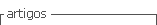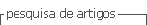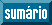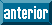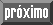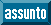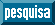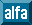## Artigo

•Citado por SciELO
•Acessos

•Citado por Google
•Similares em SciELO
•Similares em Google

## versão impressa ISSN 0122-5383

#### Resumo

ABREO, Sergio-Alberto; RAMIREZ, Ana-B  e  REYES, Oscar-Mauricio. A GPU IMPLEMENTATION OF THE SECOND ORDER ADJOINT STATE THEORY TO QUANTIFY THE UNCERTAINTY ON FWI. C.T.F Cienc. Tecnol. Futuro [online]. 2018, vol.8, n.2, pp.99-111. ISSN 0122-5383.  http://dx.doi.org/10.29047/01225383.86.

The second order scattering information provided by the Hessian matrix and its inverse plays an important role in both, parametric inversion and uncertainty quantification. On the one hand, for parameter inversion, the Hessian guides the descent direction such that the cost function minimum is reached with less iterations. On the other hand, it provides a posteriori information of the probability distribution of the parameters obtained after full waveform inversion, as a function of the a priori probability distribution information.

Nevertheless, the computational cost of the Hessian matrix represents the main obstacle in the state-of-the-art for practical use of this matrix from synthetic or real data. The second order adjoint state theory provides a strategy to compute the exact Hessian matrix, reducing its computational cost, because every column of the matrix can be obtained by performing two forward and two backward propagations.

In this paper, we first describe an approach to compute the exact Hessian matrix for the acoustic wave equation with constant density. We then provide an analysis of the use of the Hessian matrix for uncertainty quantification of the full waveform inversion of the velocity model for a synthetic example, using the 2D acoustic and isotropic wave equation operator in time.

Palavras-chave : Inverse theory; Waveform inversion; Numerical modelling.

· resumo em Espanhol     · texto em Inglês     · Inglês (pdf )